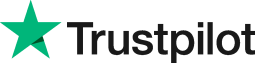Difference between two times in Excel
• Home
• Courses
• Promotions
• Schedule
• Formats
• Our Clients

Forum home » Topics » Difference between two times in Excel

# Difference between two times in Excel

Excel can carry out calculations using date and time information in order to find the difference between two times.

It does this by storing the time as a decimal of the serial number for the 1 January 1900. For example the time 12:00 midday would be stored as 0.5.

To enter times so they use the serial number, type the hours and minutes separated by a colon using the 24 hour clock (eg 13:30).

To subtract times create a formula:

= later time - earlier time

Note that Excel does not normally display negative times because that would take the date before 1 January 1900. Excel just displays a series of hashes as the result in the cell.

But suppose in a project you wish to calculate:

Actual time - Planned time

A way to display the time as a negative is to use the 1904 starting date system. In Excel 2010, select File, Options, Advanced. From the When Calculating this Workbook section, tick Use 1904 Date System.

The only issue with doing this is if your workbook is linked to another workbook using the 1900 date system, then the dates retrieved by the link would be incorrect. Otherwise the negative time calculations would be correct.

# Related forum posts:

## Excel - time calculations

Hi there, I am trying to replicate a timesheet that we used in a previous role where the formula could calculate hours due/owed by the employee. I have managed to get the formula to work as long as the employee does their hours or over time but it will not show a negative result in the event that an employee does not do enough for that day. At present the calculation is relatively simple with a cell which adds up the finish time minus the start time and time taken for lunch (hours actual

## Date and times in Excel

d like to understand, my helpdesk system- Supportworks (Hornbill), gives m daes through of when a call was opened and when a cll was fixed, I need to work out if a call met SLA whic is typically an 8hr fix (on-Fiday, 9 am > 5.30pm), so I need to work out a formula which will how this

# Related articles

## How Are Dates Stored In Excel?

Have you ever wondered how a date is actually stored in Excel? This article describes how Excel stores dates and covers an example of how this can be used in calculations.

 Welcome. Please choose your application (eg. Excel) and then post your question. Our Microsoft Qualified trainers will then respond within 24 hours (working days). Frequently Asked Questions What does 'Resolved' mean? Any suggestions, questions or comments? Please post in the Improve the forum thread.TrustScore 4.8 / 5       1238 reviews